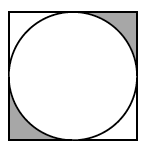### Home > CCG > Chapter 9 > Lesson 9.1.1 > Problem9-10

9-10.

The diagram at right shows a circle inscribed in a square. Find the area of the shaded region if the side length of the square is $6$ meters.Find the area of the square.

Find the area of the circle. Remember that the area of a circle is $πr²$.

Subtract the area of the circle from the area of the square.

Divide this area by two to find the area of two corners.